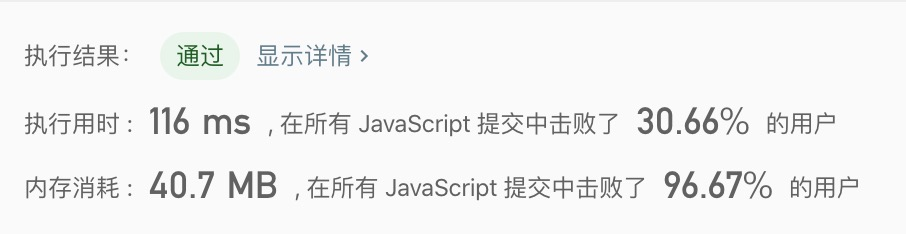Time Flying### 143.Reorder List

Given a singly linked list L: L0 → L1 → .. → Ln-1 → Ln, reorder it to: L0 → Ln → L1 → Ln-1 → L2 → Ln-2 →

You may not modify the values in the list's nodes, only nodes itself may be changed.

Example 1:

Given 1->2->3->4, reorder it to 1->4->2->3.

Example 2:

Given 1->2->3->4->5, reorder it to 1->5->2->4->3.

### Analyze

1. 步骤一: 找到链表中点后分割其为 left 链表、right 链表两部分;
2. 步骤二: 翻转 right 链表, 翻转链表思路同 206.Reverse_Linked_List;
3. 步骤三: 接着从 left 链表的左侧, 翻转后的 right 链表的左侧各取一个值进行交替拼接;

s               qdummy -> 1 -> 2 -> 3 -> 4 -> 5 -> NULL

l1 -> 2 -> 3 -> null
r4 -> 5 -> NULL

l1 -> 2 -> 3 -> null
r5 -> 4 -> NULL

l1 -> 5 -> 2 -> 3 -> NULL
r4 -> NULL

cur  next  1 -> 2 -> 3 -> null
prev curnull <- 1 <- 2 -> 3 -> null
prev curnull <- 1 <- 2 <- 3 -> null
prevnull <- 1 <- 2 <- 3 -> null
/** * Definition for singly-linked list. * function ListNode(val) { *     this.val = val; *     this.next = null; * } *//** * @param {ListNode} head * @return {void} Do not return anything, modify head in-place instead. */var reorderList = function(head) {  const dummy = new ListNode(0)  dummy.next = head
let slow = dummy  let quick = dummy
while (quick && quick.next) {    slow = slow.next    quick = quick.next    quick = quick.next  }
let right = slow.next  slow.next = null  let left = dummy.next
right = reverseList(right)
while (left && right) {    let lNext = left.next    let rNext = right.next    right.next = left.next    left.next = right    left = lNext    right = rNext  }
return dummy.next}
var reverseList = (list) => {  let prev = null  let cur = list
while (cur) {    let next = cur.next    cur.next = prev
prev = cur    cur = next  }
return prev}148(快慢指针)、234